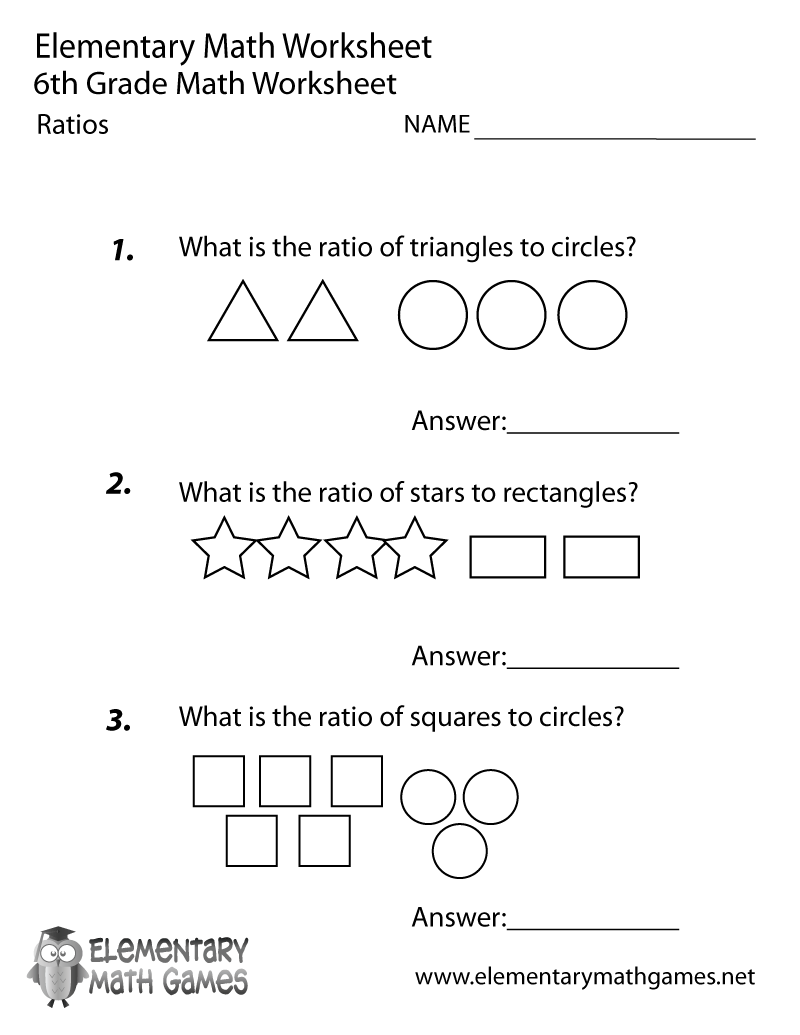# Sixth Grade Ratios Worksheet## Learn and Practice Ratios

This sixth grade math worksheet is a great way for students to learn about ratios. Sixth graders have to figure out the ratio of triangles to circles, stars to rectangles, and squares to circles.

Here are the two versions of this free sixth grade math worksheet: